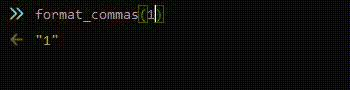<= Get me outta here!

By jetstream0/Prussia | 15/11/2022 |

I had to add commas to a number in Javascript today. I thought it was kinda interesting, and here is what I came up with:

function format_commas(amount) {
let amount_mod = String(amount);
//iterate the amount of commas there are
for (let i=0; i < Math.floor((String(amount).length-1)/3); i++) {
let position = amount_mod.length-3*(i+1)-i;
amount_mod = amount_mod.substring(0, position)+","+amount_mod.substring(position, amount_mod.length);
}
return amount_mod;
}

Basically, we calculate how many commas we will need to add (`Math.floor((String(amount).length-1)/3)`). If the `amount` is 3 digits, we need 0 commas, since `floor((3-1)/3) = floor(2/3) = 0`. If the `amount` is 7 digits, we need 2 commas, since `floor((7-1)/3) = floor(6/3) = 2`. And so on.

Then, we do a for loop with that number, and insert our commas, starting from the back. We find the position where we need to split the string in half, and then insert a comma in between the two halves of the string.

I think the most interesting part of this code was the 5th line (`let position = amount_mod.length-3*(i+1)-i;`). You might be wondering with the `-i` at the end is necessary. That's there because we are increasing the string's length by adding a comma, so we need to offset it. Remember, we are inserting commas starting from the back of the string, so we are subtracting to offset, not adding.Here is a version that can handle decimals (keep in mind that Javascript does cut off decimals after a certain point), negative numbers and invalid inputs:

function format_commas(amount) {
if (isNaN(Number(amount))) {
return amount;
}
let negative = amount < 0;
amount = Math.abs(amount);
let before_dec = String(amount).split('.');
let amount_mod = before_dec;
//iterate the amount of commas there are
for (let i=0; i < Math.floor((before_dec.length-1)/3); i++) {
let position = amount_mod.length-3*(i+1)-i;
amount_mod = amount_mod.substring(0, position)+","+amount_mod.substring(position, amount_mod.length);
}
if (String(amount).split('.')) {
amount_mod = amount_mod+"."+String(amount).split('.');
}
if (negative) {
amount_mod = `-\${amount_mod}`;
}
return amount_mod;
}

`I had to add commas to a number in Javascript today. I thought it was kinda interesting, and here is what I came up with:`

````js`

`function format_commas(amount) {`

`  let amount_mod = String(amount);`

`  //iterate the amount of commas there are`

`  for (let i=0; i < Math.floor((String(amount).length-1)/3); i++) {`

`    let position = amount_mod.length-3*(i+1)-i;`

`    amount_mod = amount_mod.substring(0, position)+","+amount_mod.substring(position, amount_mod.length);`

`  }`

`  return amount_mod;`

`}`

`````

`Basically, we calculate how many commas we will need to add (`Math.floor((String(amount).length-1)/3)`). If the `amount` is 3 digits, we need 0 commas, since `floor((3-1)/3) = floor(2/3) = 0`. If the `amount` is 7 digits, we need 2 commas, since `floor((7-1)/3) = floor(6/3) = 2`. And so on.`

`Then, we do a for loop with that number, and insert our commas, *starting from the back*. We find the position where we need to split the string in half, and then insert a comma in between the two halves of the string.`

`I think the most interesting part of this code was the 5th line (`let position = amount_mod.length-3*(i+1)-i;`). You might be wondering with the `-i` at the end is necessary. That's there because we are increasing the string's length by adding a comma, so we need to offset it. Remember, we are inserting commas starting from the back of the string, so we are subtracting to offset, not adding.`

`![Demo](/images/commas.gif)`

`Here is a version that can handle decimals (keep in mind that Javascript does cut off decimals after a certain point), negative numbers and invalid inputs:`

````js`

`function format_commas(amount) {`

`  if (isNaN(Number(amount))) {`

`    return amount;`

`  }`

`  let negative = amount < 0;`

`  amount = Math.abs(amount);`

`  let before_dec = String(amount).split('.');`

`  let amount_mod = before_dec;`

`  //iterate the amount of commas there are`

`  for (let i=0; i < Math.floor((before_dec.length-1)/3); i++) {`

`    let position = amount_mod.length-3*(i+1)-i;`

`    amount_mod = amount_mod.substring(0, position)+","+amount_mod.substring(position, amount_mod.length);`

`  }`

`  if (String(amount).split('.')) {`

`    amount_mod = amount_mod+"."+String(amount).split('.');`

`  }`

`  if (negative) {`

`    amount_mod = `-\${amount_mod}`;`

`  }`

`  return amount_mod;`

`}`

`````